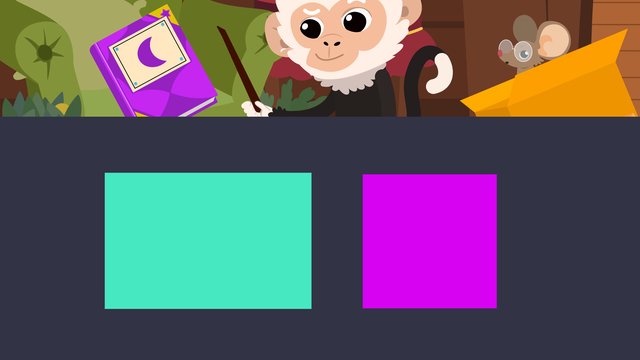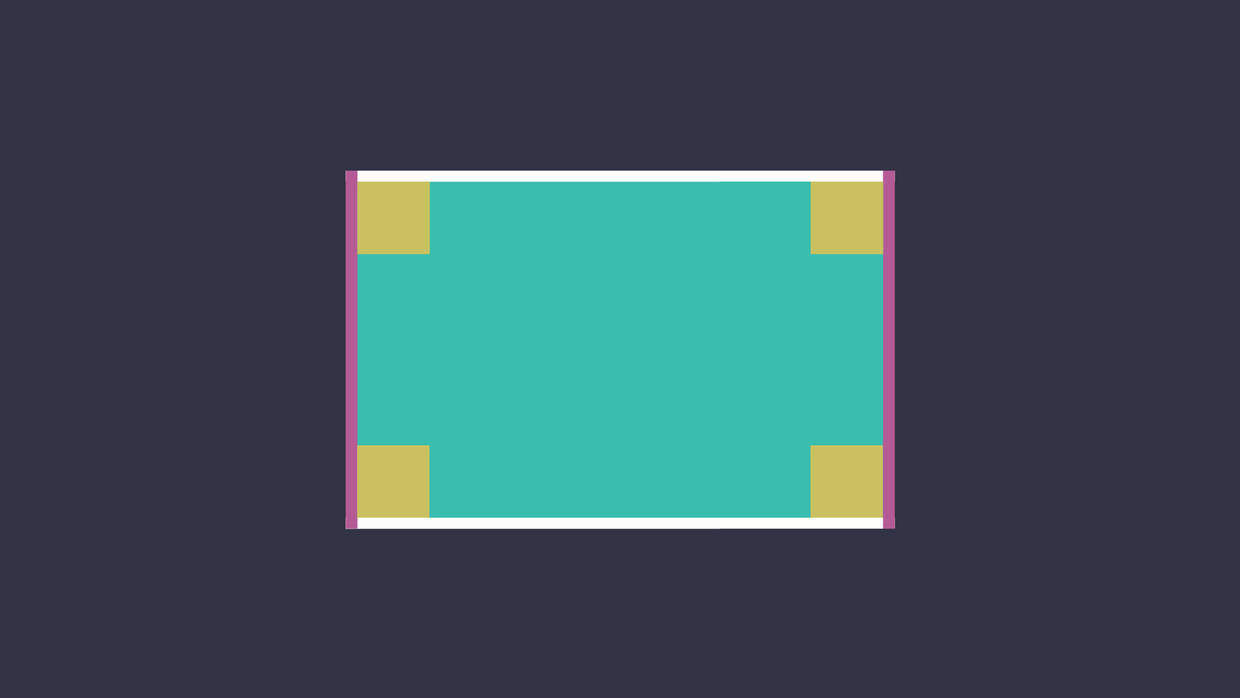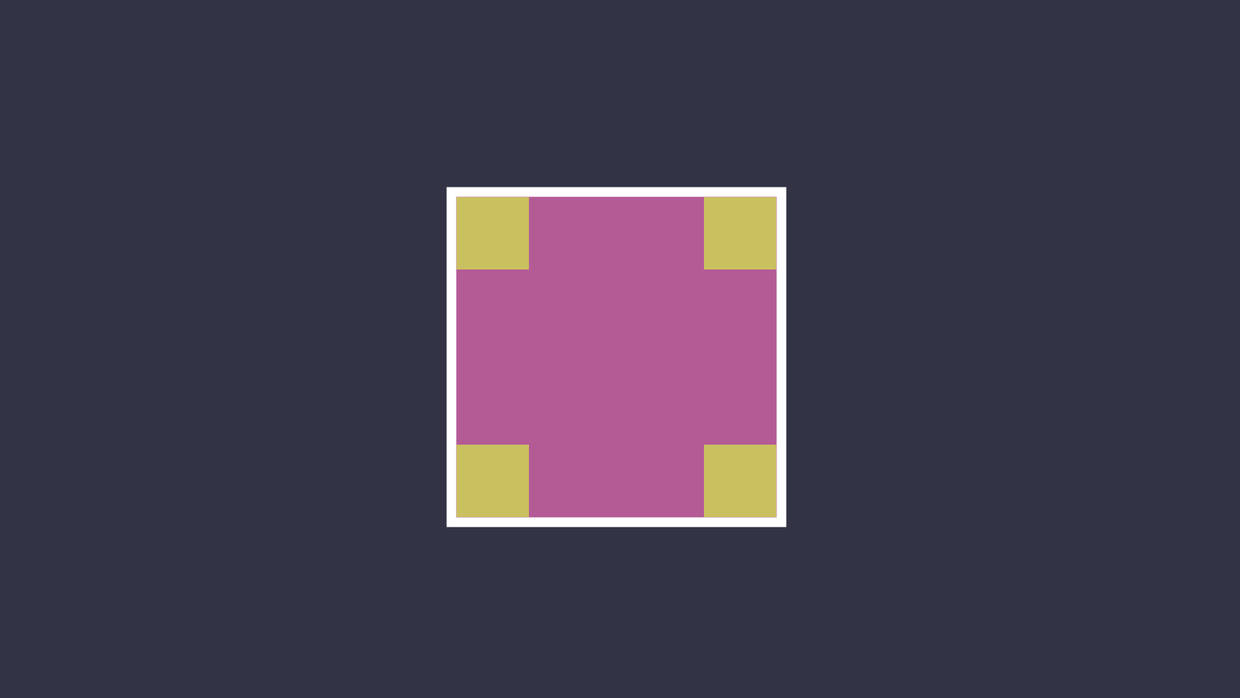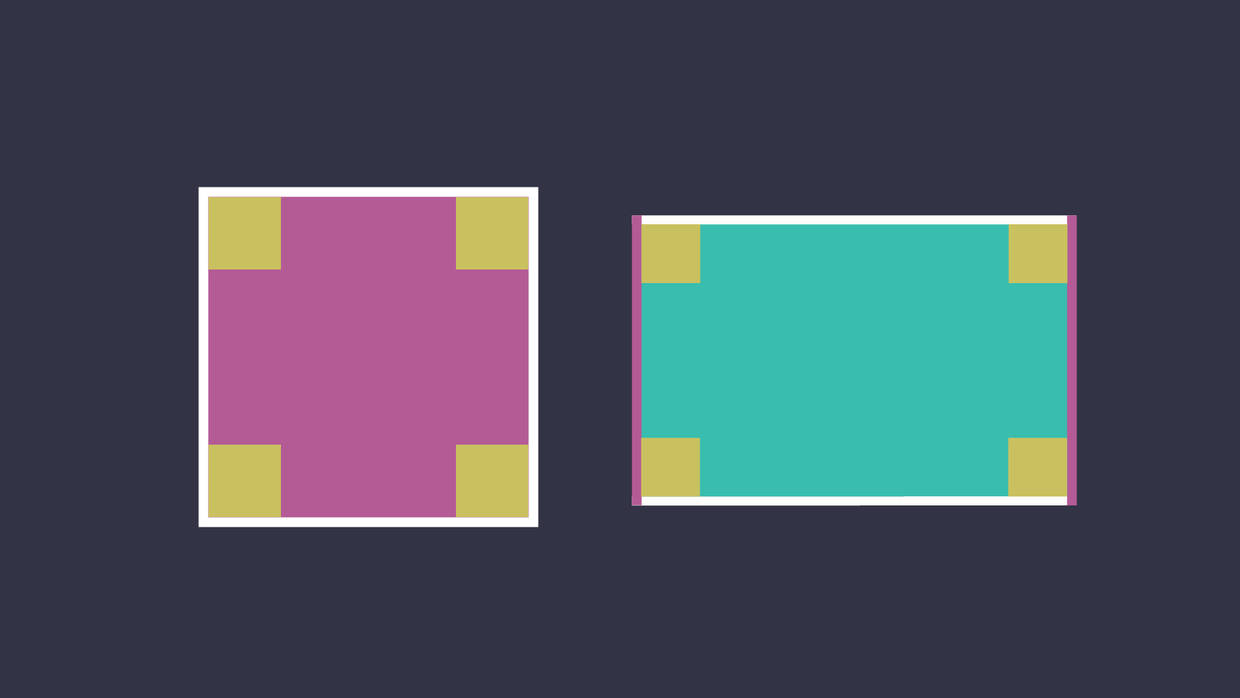# Quadrilaterals – Rectangles and SquaresRate this video

Ø 5.0 / 1 ratings

The authorTeam Digital

## DescriptionQuadrilaterals – Rectangles and Squares

### How to Identify a Rectangle and a Square

Today, Nico is a wizard and he finds a spell book on his chair. In order to produce the magic spells inside, Nico needs to learn about quadrilaterals, especially rectangles and squares. They learn the properties of rectangles and squares, and Nico is able to produce some magic! The first spell works so well, that Nico excitedly rushes to conduct the next spell, and spills water all over Nia!

In this video, you will learn the definition of a rectangle in geometry. You will also learn the definition of a square too.

A quadrilateral is a polygon with four straight sides. Any four sided shape you can think of is a quadrilateral. Is a rectangle shape a polygon that is a quadrilateral? Yes, it is! What about a square? It has four sides, so it is a quadrilateral too. Rectangles and squares are both a type of quadrilateral!

Quadrilaterals are Everywhere! Can you see any around you? A window, or the front of your TV are great examples of quadrilaterals.

Shapes can be identified by their attributes, or properties, as you will see below!

### What is a Rectangle?

Rectangle definition: A rectangle is a polygon that has four straight sides.

It is a quadrilateral because it has four sides. How many vertices does a rectangle have? The sides of a rectangle meet at a 90 degree angle, known as a right angle, and there are four of them. How many pairs of parallel sides does a rectangle have? A rectangle has two pairs of parallel sides that are equal in length.

What does a rectangle look like?

Below you can see a rectangle outline.#### Properties of a Rectangle

• Two pairs of opposite sides equal in length
• Four right angles

### What is a Square?

Square definition: A square is a polygon that has four straight sides that are all equal in length.

It is a quadrilateral because it has four sides. How many vertices does a rectangle have? The sides of a square meet at a 90 degree angle, known as a right angle, and there are four of them. How many pairs of parallel sides does a square have? A square also has two pairs of parallel sides that are all equal in length.

What does a square look like?

Below you can see a square outline.#### Properties of a Square

• Two pairs of opposite sides all equal in length
• Four right angles

### Summary of Rectangles and SquaresRectangles and squares are both shapes that are quadrilaterals. Both shapes have four straight sides and four right angles. While they are similar in properties, the main difference between a rectangle and a square is that a square has sides that are all in length.

## Quadrilaterals – Rectangles and Squares Exercise

Would you like to practice what you’ve just learned? Practice problems for this video Quadrilaterals – Rectangles and Squares help you practice and recap your knowledge.
• ### Find the quadrilaterals in Nico's attic.

Hints

There are 5 quadrilaterals to find.

Solution
• There are 5 quadrilaterals in total: 2 squares and 3 rectangles.
• The rug, broom, blanket, and glasses are not quadrilaterals because they aren't polygons with 4 straight sides.
• ### Describe the different quadrilateral shapes.

Hints

Here are some examples of quadrilaterals.

Solution

A quadrilateral is a polygon with 4 straight sides. We learned that squares and rectangles are different types of quadrilaterals.

Squares are quadrilaterals with 4 equal sides and 4 right angles. Rectangles are quadrilaterals with 2 pairs of opposite sides that are equal and 4 right angles. Every square is a special type of rectangle because it has 2 pairs of opposite sides that are equal in length.

• ### Identify the squares.

Hints

Make sure your shape is a quadrilateral that has 4 right angles.

Make sure your shape has 4 equal sides.

There are 3 squares.

Solution

The window, purple shape, and orange shape are all squares because they are all quadrilaterals that have 4 right angles and 4 equal sides.

The rest are all not squares.
The first shape is a rectangle because it has 2 pairs of equal sides.
The second shape is not a square because it isn't enclosed, therefore isn't a polygon.
The third shape is not a square because it doesn't have 4 right angles.
The last shape is a rectangle because it has 2 pairs of equal sides.

• ### How many rectangles do you see?

Hints

Remember rectangles are quadrilaterals that have 2 pairs of equal sides and 4 right angles.

Look at the shapes that might be rotated.

Remember squares are a special type of rectangle.

Solution

There are 4 rectangles. 2 are special rectangles that are squares.
All 4 shapes have 2 pairs of equal sides and 4 right angles.

Hints

Solution

The square and rectangle are quadrilaterals because they both are polygons with 4 straight sides.

• ### Sort the shapes.

Hints

Remember rectangles are quadrilaterals with 2 pairs of equal sides and 4 right angles.

Squares are both a square and also a special rectangle.

Solution

The squares are special rectangles, so they are both a rectangle and a square.
The rectangles have 2 pairs of equal sides and 4 right angles.
The triangle and parallelogram are neither a square or a rectangle.